upm  1.7.1 Sensor/Actuator repository for libmraa (v2.0.0)
LSM303AGR Class Reference

API for the LSM303AGR 3-Axis Geomagnetic Sensor. More...

## Detailed Description

The LSM303AGR is an ultra-low-power high-performance system-in-package featuring a 3D digital linear acceleration sensor and a 3D digital magnetic sensor. The LSM303AGR has linear acceleration full scales of 2g/4g/8g/16g and a magnetic field dynamic range of 50 Gauss.

Not all functionality of this chip has been implemented in this driver, however all the pieces are present to add any desired functionality. This driver supports only I2C operation.

This device requires 3.3v operation.

// Instantiate an LSM303AGR using default I2C parameters
// now output data every 250 milliseconds
while (shouldRun) {
float x, y, z;
sensor.update();
sensor.getAccelerometer(&x, &y, &z);
cout << "Accelerometer x: " << x << " y: " << y << " z: " << z << " g" << endl;
sensor.getMagnetometer(&x, &y, &z);
cout << "Magnetometer x: " << x << " y: " << y << " z: " << z << " uT" << endl;
cout << "Temperature: " << sensor.getTemperature() << " C" << endl;
cout << endl;
upm_delay_us(250000);
}

## Public Member Functions

~LSM303AGR ()

void update ()

void getMagnetometer (float *x, float *y, float *z)

std::vector< float > getMagnetometer ()

void getAccelerometer (float *x, float *y, float *z)

std::vector< float > getAccelerometer ()

float getTemperature ()

void init (LSM303AGR_POWER_MODE_T usage=LSM303AGR_POWER_HIGH_RESOLUTION)

void setFullScale (LSM303AGR_A_FS_T fs)

void setPowerMode (LSM303AGR_POWER_MODE_T mode)

void setAccelerometerODR (LSM303AGR_A_ODR_T odr)

void setMagnetometerODR (LSM303AGR_CFG_A_M_ODR_T odr)

uint8_t getAccelerometerInt1Config ()

uint8_t getAccelerometerInt2Config ()

void setAccelerometerInt1Config (uint8_t bits)

void setAccelerometerInt2Config (uint8_t bits)

uint8_t getMagnetometerIntConfig ()

void setMagnetometerIntConfig (uint8_t bits)

uint8_t getAccelerometerInt1Src ()

uint8_t getAccelerometerInt2Src ()

uint8_t getMagnetometerIntSrc ()

void installISR (LSM303AGR_INTERRUPT_PINS_T intr, int gpio, mraa::Edge level, void(*isr)(void *), void *arg)

void uninstallISR (LSM303AGR_INTERRUPT_PINS_T intr)

## Protected Member Functions

uint8_t readReg (uint8_t reg)

int readRegs (uint8_t reg, uint8_t *buffer, int len)

void writeReg (uint8_t reg, uint8_t val)

## Protected Attributes

lsm303agr_context m_lsm303agr

## Constructor & Destructor Documentation

 LSM303AGR ( int bus = `LSM303AGR_DEFAULT_I2C_BUS`, int acc_addr = `LSM303AGR_DEFAULT_ACC_ADDR`, int mag_addr = `LSM303AGR_DEFAULT_MAG_ADDR` )

LSM303AGR constructor

This driver can only support I2C. SPI requires support for 3-wire SPI which we cannot currently handle. Only the basic capabilities of the device are supported, however there is a full register map defined (lsm303agr_defs.h), and with access to the bus read/write functions, any desired additional functionality can be implemented.

Parameters
 bus I2C bus to use acc_addr The I2C address of the accelerometer. Use -1 if you do not wish to use the accelerometer. mag_addr The I2C address of the magnetometer. Use -1 if you do not wish to use the magnetometer.
Returns
The device context, or NULL if an error occurred
Exceptions
 std::runtime_error on failure.
 ~LSM303AGR ( )

LSM303AGR Destructor

Here is the call graph for this function: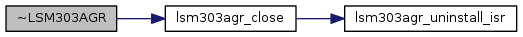## Member Function Documentation

 void update ( void )

Update the internal stored values from sensor data. This method must be called before querying the acceleration, magnetometer, or temperature.

Exceptions
 std::runtime_error on failure

Here is the call graph for this function: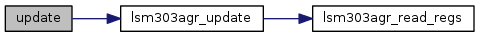void getMagnetometer ( float * x, float * y, float * z )

Return magnetometer data in micro-Teslas (uT). update() must have been called prior to calling this method.

Parameters
 x Pointer to a floating point value that will have the current x component placed into it y Pointer to a floating point value that will have the current y component placed into it z Pointer to a floating point value that will have the current z component placed into it

Here is the call graph for this function: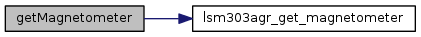std::vector< float > getMagnetometer ( )

Return magnetometer data in micro-Teslas (uT) in the form of a floating point vector. update() must have been called prior to calling this method.

Returns
A floating point vector containing x, y, and z in that order
 void getAccelerometer ( float * x, float * y, float * z )

Return acceleration data in gravities. update() must have been called prior to calling this method.

Parameters
 x Pointer to a floating point value that will have the current x component placed into it y Pointer to a floating point value that will have the current y component placed into it z Pointer to a floating point value that will have the current z component placed into it

Here is the call graph for this function: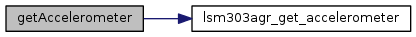std::vector< float > getAccelerometer ( )

Return acceleration data in gravities in the form of a floating point vector. update() must have been called prior to calling this method.

Returns
A floating point vector containing x, y, and z in that order
 float getTemperature ( void )

Return temperature data in degrees Celsius. NOTE: This is not the ambient room temperature. update() must have been called prior to calling this method.

Returns
Temperature in degrees Celsius

Here is the call graph for this function: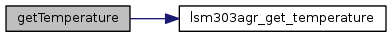void init ( LSM303AGR_POWER_MODE_T usage = `LSM303AGR_POWER_HIGH_RESOLUTION` )

Initialize the device and start operation. This function is called from the constructor so it will not typically need to be called by a user unless the device is reset. It will initialize the accelerometer and magnetometer (if enabled) to certain default running modes.

For the accelerometer, the full scale will be set to 2G, mode continuous, all axes enabled, BDU enabled, temperature measurement enabled, and an output data rate (ODR) of 100Hz with the power mode set at high resolution.

For the magnetometer, temperature compensation will be enabled, mode continuous, and an output data rate of 10Hz with the power mode set at high resolution.

Parameters
 usage One of the LSM303AGR_POWER_MODE_T values. The default is LSM303AGR_POWER_HIGH_RESOLUTION.
Exceptions
 std::runtime_error on failure

Here is the call graph for this function: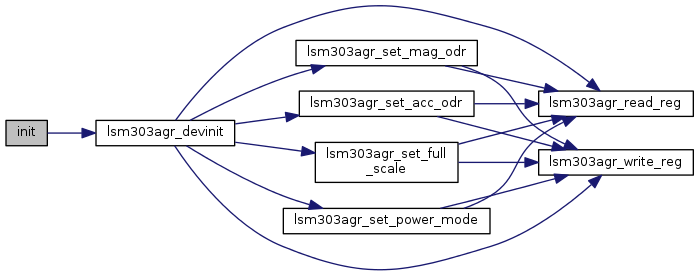void setFullScale ( LSM303AGR_A_FS_T fs )

Set the full scale (sensitivity) value for the accelerometer. This device supports 2G, 4G, 8G, and 16G full scale modes.

Parameters
 fs One of the LSM303AGR_A_FS_T values
Exceptions
 std::runtime_error on failure

Here is the call graph for this function:void setPowerMode ( LSM303AGR_POWER_MODE_T mode )

Set an operating power mode. There are 3 modes available: low power, normal, and high resolution.

Parameters
 mode One of the LSM303AGR_POWER_MODE_T values. The default set at initialization time is LSM303AGR_POWER_HIGH_RESOLUTION.
Exceptions
 std::runtime_error on failure

Here is the call graph for this function:void setAccelerometerODR ( LSM303AGR_A_ODR_T odr )

Set the accelerometer output data rate (ODR)

Parameters
 odr One of the LSM303AGR_A_ODR_T values. The default set at initialization time is LSM303AGR_A_ODR_100HZ.
Exceptions
 std::runtime_error on failure

Here is the call graph for this function:void setMagnetometerODR ( LSM303AGR_CFG_A_M_ODR_T odr )

Set the magnetometer output data rate (ODR)

Parameters
 odr One of the LSM303AGR_CFG_A_M_ODR_T values. The default set at initialization time is LSM303AGR_CFG_A_M_ODR_10HZ.
Exceptions
 std::runtime_error on failure

Here is the call graph for this function:uint8_t getAccelerometerInt1Config ( )

Return the accelerometer interrupt 1 config register. This register allows you to enable various interrupt conditions. See the datasheet for details.

Returns
A bitmask of LSM303AGR_INT_CFG_A_BITS_T bits

Here is the call graph for this function: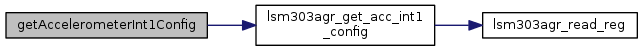uint8_t getAccelerometerInt2Config ( )

Return the accelerometer interrupt 2 config register. This register allows you to enable various interrupt conditions. See the datasheet for details.

Returns
A bitmask of LSM303AGR_INT_CFG_A_BITS_T bits

Here is the call graph for this function: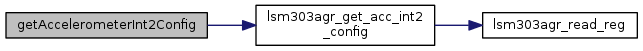void setAccelerometerInt1Config ( uint8_t bits )

Set the accelerometer interrupt 1 config register. See the datasheet for details.

Parameters
 dev The device context bits A bitmask of LSM303AGR_INT_CFG_A_BITS_T bits
Exceptions
 std::runtime_error on failure

Here is the call graph for this function: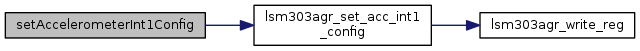void setAccelerometerInt2Config ( uint8_t bits )

Set the accelerometer interrupt 2 config register. See the datasheet for details.

Parameters
 dev The device context bits A bitmask of LSM303AGR_INT_CFG_A_BITS_T bits
Exceptions
 std::runtime_error on failure

Here is the call graph for this function: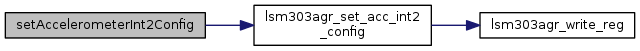uint8_t getMagnetometerIntConfig ( )

Return the magnetometer interrupt config register. See the datasheet for details.

Returns
A bitmask of LSM303AGR_INT_CTRL_REG_M_BITS_T bits

Here is the call graph for this function: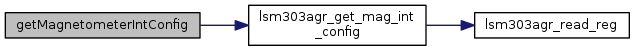void setMagnetometerIntConfig ( uint8_t bits )

Set the magnetometer (mag) interrupt config register. See the datasheet for details.

Parameters
 bits A bitmask of LSM303AGR_INT_CTRL_REG_M_BITS_T bits
Exceptions
 std::runtime_error on failure

Here is the call graph for this function: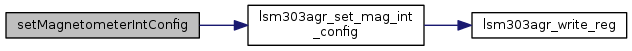uint8_t getAccelerometerInt1Src ( )

Return the accelerometer interrupt 1 source register. This register indicates which interrupts have been triggered. See the datasheet for details.

Returns
a bitmask of LSM303AGR_INT_SRC_A_BITS_T bits

Here is the call graph for this function: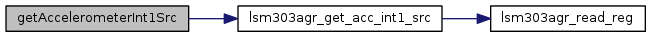uint8_t getAccelerometerInt2Src ( )

Return the accelerometer interrupt 2 source register. This register indicates which interrupts have been triggered. See the datasheet for details.

Returns
a bitmask of LSM303AGR_INT_SRC_A_BITS_T bits

Here is the call graph for this function: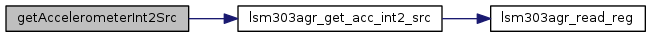uint8_t getMagnetometerIntSrc ( )

Return the magnetometer (mag) interrupt source register. This register indicates which interrupts have been triggered. See the datasheet for details.

Parameters
 dev The device context
Returns
a bitmask of LSM303AGR_INT_SRC_REG_M_BITS_T bits

Here is the call graph for this function: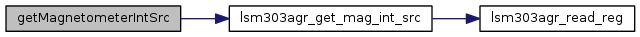void installISR ( LSM303AGR_INTERRUPT_PINS_T intr, int gpio, mraa::Edge level, void(*)(void *) isr, void * arg )

Install an interrupt handler

Parameters
 intr One of the LSM303AGR_INTERRUPT_PINS_T values specifying which interrupt pin you are installing gpio GPIO pin to use as interrupt pin level The interrupt trigger level (one of mraa::Edge values). Make sure that you have configured the interrupt pin properly for whatever level you choose. isr The interrupt handler, accepting a void * argument arg The argument to pass the the interrupt handler
Exceptions
 std::runtime_error on failure

Here is the call graph for this function: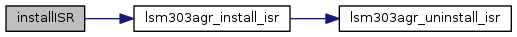void uninstallISR ( LSM303AGR_INTERRUPT_PINS_T intr )

Uninstall a previously installed interrupt handler

Parameters
 intr One of the LSM303AGR_INTERRUPT_PINS_T values specifying which interrupt pin you are removing

Here is the call graph for this function: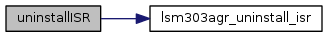uint8_t readReg ( uint8_t reg )
protected

Parameters
 reg The register to read
Returns
The value of the register

Here is the call graph for this function: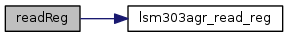int readRegs ( uint8_t reg, uint8_t * buffer, int len )
protected

Read contiguous registers into a buffer

Parameters
 buffer The buffer to store the results len The number of registers to read
Returns
The number of bytes read
Exceptions
 std::runtime_error on failure

Here is the call graph for this function: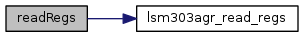void writeReg ( uint8_t reg, uint8_t val )
protected

Write to a register

Parameters
 reg The register to write to val The value to write
Exceptions
 std::runtime_error on failure

Here is the call graph for this function: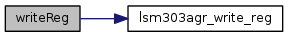Collaboration diagram for LSM303AGR: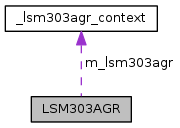[legend]

The documentation for this class was generated from the following files: